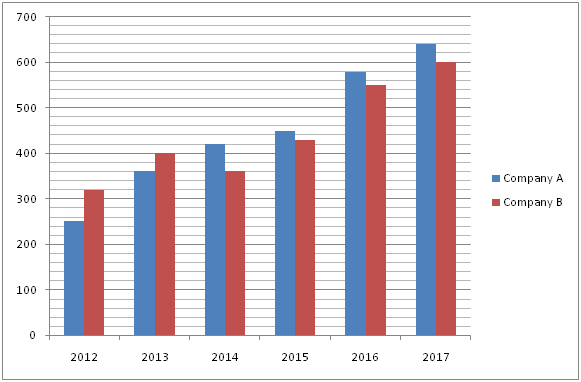# LIC AAO/SBI PO Prelims Quantitative Aptitude Questions 2019 (Day-14)

Dear Aspirants, Our IBPS Guide team is providing new series of Quantitative Aptitude Questions for LIC AAO/SBI PO 2019 so the aspirants can practice it on a daily basis. These questions are framed by our skilled experts after understanding your needs thoroughly. Aspirants can practice these new series questions daily to familiarize with the exact exam pattern and make your preparation effective.

[WpProQuiz 5715]

### Click Here for SBI PO Pre 2019 High-Quality Mocks Exactly on SBI Standard

Directions (1 – 5): What value should come in place of question mark (?) in the following questions?

1) 45 × 270 ÷ 9 + ? – 4900 = 256 ÷ 8 + 122

a) 3726

b) 3850

c) 2538

d) 2962

e) None of these

2) 3 × 36 + 32% of 4500 – 26 % of 1300 =?

a) 1860

b) 1655

c) 1210

d) 1435

e) None of these

3) (1.21)4 ÷ (1331 ÷ 1000)3 × (0.11 × 10)3 = (1.1)? – 2

a) 7

b) 5

c) 6

d) 4

e) None of these

4) 42 % of 1800 – 350 ÷ 14 + 143 – (5/9) of 135 = ?

a) 1850

b) 3400

c) 3250

d) 1700

e) None of these

5) 3 (4/7) + 5 (3/14) – 4 (½) + 6 (1/14) = ? – 2 (1/7)

a) 13 (3/7)

b) 11 (5/7)

c) 12 (½)

d) 10 (3/14)

e) None of these

Directions (6 – 10): Study the following information carefully and answer the given questions:

The following bar graph shows the number of projects handled by 2 different companies in 6 different years.6) Find the difference between the total number of projects handled by company A in the year 2013, 2015 and 2017 together and total number of projects handled by company B in the year 2012, 2014 and 2016 together.

a) 360

b) 220

c) 280

d) 450

e) None of these

7) Total number of projects handled by both the companies together in the year 2014 is approximately what percentage of total number of projects handled by both the companies together in the year 2016?

a) 78 %

b) 85 %

c) 96 %

d) 69 %

e) 53 %

8) Find the average number of projects handled by company A in all the given years together.

a) 450

b) 380

c) 290

d) 420

e) None of these

9) Find the ratio between the total number of projects handled by both the companies together in the year 2012 to that of 2017.

a) 49: 133

b) 28: 117

c) 57: 124

d) 32: 99

e) None of these

10) Find the difference between the average number of projects handled by company A in the year 2012, 2014 and 2016 together to that of the average number of projects handled by company B in the year 2013, 2015 and 2017 together.

a) 45

b) 70

c) 75

d) 60

e) None of these

Direction (1-5) :

45 × 270 ÷ 9 + x – 4900 = 256 ÷ 8 + 122

1350 + x – 4900 = 32 + 144

x = 32 + 144 + 4900 – 1350

x = 3726

3 × 36 + 32% of 4500 – 26 % of 1300 = x

x = 108 + 1440 – 338 = 1210

(1.21)4 ÷ (1331 ÷ 1000)3 × (0.11 × 10)3 = (1.1)x – 2

((1.1)2)4 ÷ (1.1)9 × (1.1)3 = (1.1)x– 2

(1.1)8 ÷ (1.1)9 × (1.1)3 = (1.1)x– 2

(1.1)8 – 9 + 3 = (1.1)x – 2

(1.1)2 = (1.1)x – 2

2 = x – 2

x = 4

(42/100)*1800 – (350/14) + 2744 – (5/9)*135 = x

x = 756 – 25 + 2744 – 75

x = 3400

3 (4/7) + 5 (3/14) – 4 (½) + 6 (1/14) + 2 (1/7) = x

x = (3 + 5 – 4 + 6 + 2) (4/7 + 3/14 – ½ + 1/14 + 1/7)

x = 12 [(8 + 3 – 7 + 1 + 2)/14] = 12 (7/14)

x = 12 (½)

Direction (6-10) :

The total number of projects handled by company A in the year 2013, 2015 and 2017 together

= >360 + 450 + 640 = 1450

The total number of projects handled by company B in the year 2012, 2014 and 2016 together

= >320 + 360 + 550 = 1230

Required difference = 1450 – 1230 = 220

Total number of projects handled by both the companies together in the year 2014

= >420 + 360 = 780

Total number of projects handled by both the companies together in the year 2016

= >580 + 550 = 1130

Required % = (780/1130)*100 = 69 %

The total number of projects handled by company A in all the given years together

= > 250 + 360 + 420 + 450 + 580 + 640 = 2700

Required average = 2700/6 = 450

The total number of projects handled by both the companies together in the year 2012

= >250 + 320 = 570

The total number of projects handled by both the companies together in the year 2017

= >640 + 600 = 1240

Required ratio = 570: 1240 = 57: 124

The average number of projects handled by company A in the year 2012, 2014 and 2016 together

= > (250 + 420 + 580)/3 = 1250/3

The average number of projects handled by company B in the year2013, 2015 and 2017 together

= > (400 + 430 + 600)/3 = 1430/3

Required difference = (1430 – 1250)/3 = 180/3 = 60